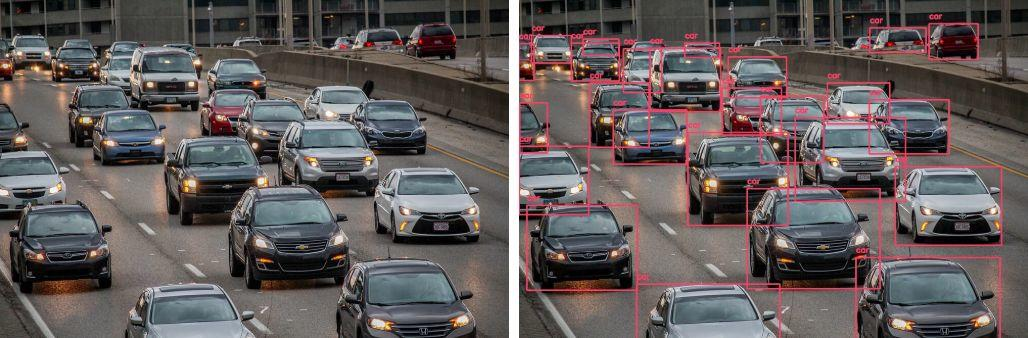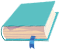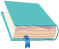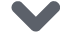# 使用Python在少于10行代码中计数汽车数量 ｜视觉实战###### 来源|Medium

Python版本 3.6.9cvlib: 0.2.2opencv-python: 4.1.1.26tensorflow: 1.14.0matplotlib: 3.1.1Keras: 2.2.5

import cv2import matplotlib.pyplot as pltimport cvlib as cvfrom cvlib.object_detection import draw_bboxim = cv2.imread('cars_4.jpeg')bbox, label, conf = cv.detect_common_objects(im)output_image = draw_bbox(im, bbox, label, conf)plt.imshow(output_image)plt.show()print('Number of cars in the image is '+ str(label.count('car')))

Number of cars in the image is 29

Number of cars in the image is 22Number of cars in the image is 25

• cvlib

: https://www.cvlib.net/?source=post_page—–2d28eebc5b11———————-欢迎扫码关注磐创AI点击下方 |  | 了解更多